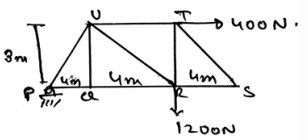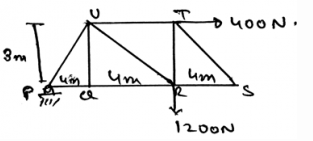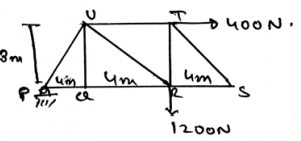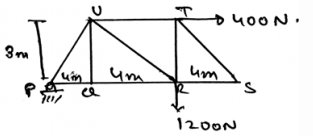Courses

# Test: Zero Force Member

## 15 Questions MCQ Test Engineering Mechanics | Test: Zero Force Member

Description
This mock test of Test: Zero Force Member for Mechanical Engineering helps you for every Mechanical Engineering entrance exam. This contains 15 Multiple Choice Questions for Mechanical Engineering Test: Zero Force Member (mcq) to study with solutions a complete question bank. The solved questions answers in this Test: Zero Force Member quiz give you a good mix of easy questions and tough questions. Mechanical Engineering students definitely take this Test: Zero Force Member exercise for a better result in the exam. You can find other Test: Zero Force Member extra questions, long questions & short questions for Mechanical Engineering on EduRev as well by searching above.
QUESTION: 1

Solution:

The zero member force are having no loadings. They doesn’t involve in the loading part of the story in the trusses. They doesn’t provide the stress nor the strain to the body of the structure. Hence they have no load associated with them.

QUESTION: 2

### The zero forces are used to improve__________ of the structure.

Solution:

The zero forces increase the stability of the structures. That the frames of the structures, which are there present in the structure. The stability of the joints, making them to be in rotational as well as translational equilibrium.

QUESTION: 3

### Find the force in the member UR.Solution:

The direction of the unknown is not known to us. To know the direction of the unknown force we take assumption of it. That is we assume that the particular direction might be the direction of the force and then we do the calculations accordingly. And then we apply the equilibrium equations to the joints.

QUESTION: 4

We find the zero force members by inspections.

Solution:

The answer is yes. We find the zero force members by inspection. We do not have a special function or any special method for finding the zero force members of the structure. But yes the zero force members are founded by inspections only.

QUESTION: 5

If two members of the structure (trusses) forms a truss joint and there is no external force acting on that joint then the two members are called___________

Solution:

If two members of the structure (trusses) forms a truss joint and there is no external force acting on that joint then the two members are called as zero force members. This is again came from inspection. If we imagine the same, we see that there are no movements, thus they are zero force members.

QUESTION: 6

If two members of the structure (trusses) form a truss joint and there is no external force acting on that joint then the two members are called___________

Solution:

If two members of the structure (trusses) forms a truss joint and there is no external force acting on that joint then the two members are called as zero force members. This is again came from inspection. If we imagine the same, we see that there are no movements, thus they are zero force members.

QUESTION: 7

If two members of the structure (trusses) forms a truss joint and there is an external force acting on that joint then the two members are called as zero force members.

Solution:

If two members of the structure (trusses) forms a truss joint and there is no external force acting on that joint then the two members are called as zero force members. This is again came from inspection. If we imagine the same, we see that there are no movements, thus they are zero force members.

QUESTION: 8

Which of following is not considered as the true condition for the three members to be zero force members?

Solution:

The three members to be having a zero member must have any two of the three members collinear. There must be no external forces acting on the section or the joint which is being selected. And obviously all the members must be attached.

QUESTION: 9

In three members having one as zero force member in truss, what if there is an external force applied?

Solution:

For making any one of the member of the truss to be as a zero member all the conditions are to be followed. Like any two of the three members collinear. There must be no external forces acting on the section or the joint which is being selected.

QUESTION: 10

What will happen if the collinearity of the two members is affected?

Solution:

For making any one of the member of the truss to be as a zero member all the conditions are to be followed. Like any two of the three members collinear. There must be no external forces acting on the section or the joint which is being selected.

QUESTION: 11

Find the force in the member QR.Solution:

The direction of the unknown is not known to us. To know the direction of the unknown force we take assumption of it. That is we assume that the particular direction might be the direction of the force and then we do the calculations accordingly. And then we apply the equilibrium equations to the joints.

QUESTION: 12

What will happen if the three members are all perpendicular to each other? No external force is applied to them.

Solution:

As far as the conditions are required for the equilibrium and for the zero force members, this joint explained will be having a zero force member. Which will be unaffected to shear stress and shear strain. Thus the zero member formation.

QUESTION: 13

Find the force in the member UT.Solution:

The direction of the unknown is not known to us. To know the direction of the unknown force we take assumption of it. That is we assume that the particular direction might be the direction of the force and then we do the calculations accordingly. And then we apply the equilibrium equations to the joints.

QUESTION: 14

The ___________ forces do not cause the rotation.

Solution:

The concurrent forces are the which are somewhere touching the axis of rotation. If any of the force is touching that axis, that force is not considered, or is insufficient to cause a rotation. If a force is concurrent then the perpendicular distance of the force from the line of axis is zero, thus no rotation. As we know rotation is caused by moment.

QUESTION: 15

Find the vertical support reactions at A.Solution:

The direction of the unknown is not known to us. To know the direction of the unknown force we take assumption of it. That is we assume that the particular direction might be the direction of the force and then we do the calculations accordingly. And then we apply the equilibrium equations to the joints.